Next: Flow Past a Spherical Up: Axisymmetric Incompressible Inviscid Flow Previous: Point Sources

# Dipole Point Sources

Consider the flow pattern generated by point source of strengthlocated on the symmetry axis at, and a point source of strength(i.e., a point sink) located on the symmetry axis at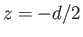. It follows, by analogy with the analysis of the previous section, that the stream function and velocity potential at a general point,, lying in the meridian plane, are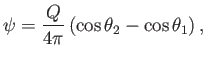(7.36)

and(7.37)

respectively. Here,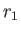,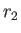,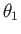, andare defined in Figure 7.2.In the limit that the product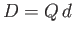remains constant, while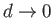, we obtain a so-called dipole point source. According to the sine rule of trigonometry,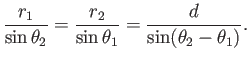(7.38)

However,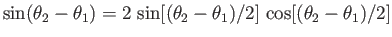, so we obtain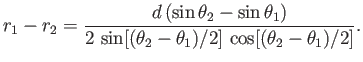(7.39)

In fact,, which leads to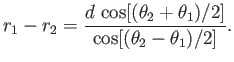(7.40)

Thus, in the limit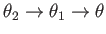and, we get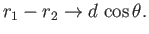(7.41)

Hence, according to Equation (7.37),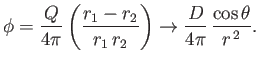(7.42)

Equation (7.36) implies that(7.43)

Thus, in the limitand, we obtain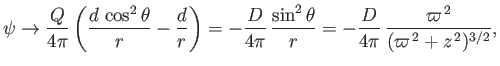(7.44)

where use has been made of Equation (7.41), as well as the fact that. Figure 7.3 shows the stream function of a dipole point source located at the origin.Incidentally, Equations (7.26), (7.28), (7.33), (7.35), (7.42), and (7.44) imply that the terms in the expansions (7.23) and (7.24) involving the constants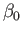,, and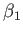correspond to a point source at the origin, uniform flow parallel to the-axis, and a dipole point source at the origin, respectively. Of course, the term involving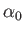is constant, and, therefore, gives rise to no flow.Next: Flow Past a Spherical Up: Axisymmetric Incompressible Inviscid Flow Previous: Point Sources
Richard Fitzpatrick 2016-03-31